Author: Oscar Cronquist Article last updated on September 25, 2018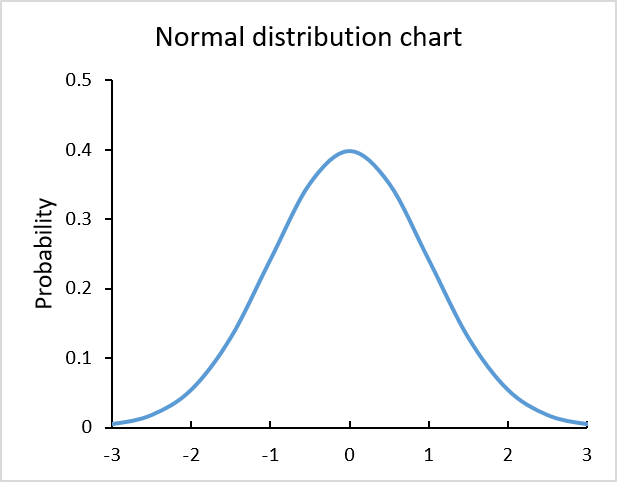The chart above is built using the NORM.DIST function and is called Normal Distribution or Bell Curve chart.

This curve is often used in probability theory and mathematical statistics.

### Instructions

First I'll show you how to construct the data needed, then insert a chart. Lastly, customize the chart to make it look better.

#### Normal distribution data

The chart requires two sets of data, x and y. Enter values from -4, -3.5, -3 ... to 4, they will be x-axis values shown in column B in the picture below.The NORM.DIST function allows you to calculate the normal distribution for each x value.

Formula in cell C21:

=NORM.DIST(B21,0,1,FALSE)

Copy and paste this formula to cells below, as far as needed.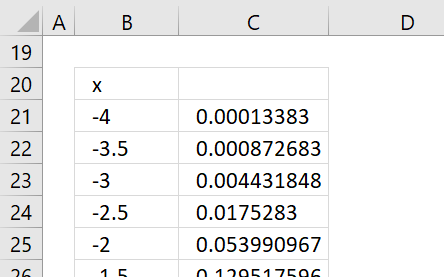#### Insert a chart

Select the cell range, in my example B21:C37.

Go to tab "Insert" on the ribbon then press with left mouse button on the scatter chart button.Press with mouse on "Scatter with smooth lines" button.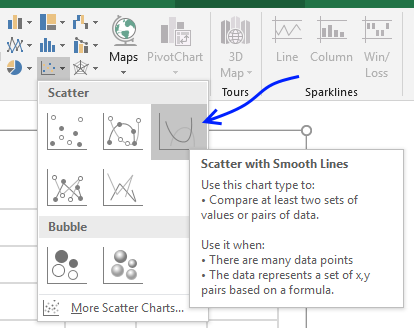The chart shows up, clearly, we have to do a few changes to this chart.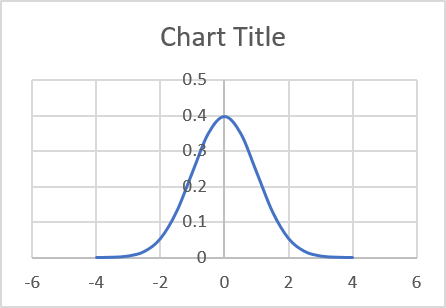#### Customize chart

The y-axis values are in the middle of the graph if you want to move it to the left or right follow these steps.

1. Press with right mouse button on on x-axis values. (Yes, x-axis.)
2. Press with mouse on "Format Axis..."
3. Find "Vertical axis crosses" setting.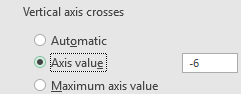4. Select Axis value and type a value. In my example, -6.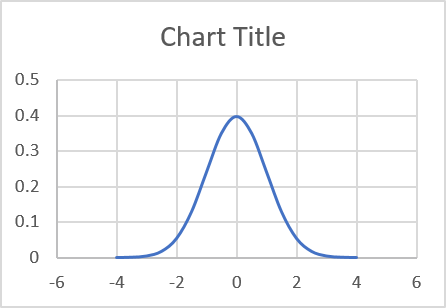Press with mouse on major gridlines and press delete button to delete them, if you prefer.

Change the chart title.

### Get Excel *.xlsx file

How to graph a normal distribution.xlsx